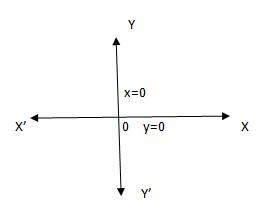Questions & AnswersQuestionAnswers

# Draw the graph of the equation x=0, y=0.Answer Verified
Hint: The equations x=0 represents y -axis and y=0 represents x –axis.

Complete step-by-step answer:
The equations given are x=0, y=0.
x=0 means to say that whatever be the value of y, the value of x would always be equal to 0
y=0 means to say that whatever be the value of x, the value of y would always be equal to 0
So, in accordance to this let us draw the graphThe origin (0,0) will be the point of intersection of the equation x=0, y=0
So, for the equation x=0, the graph would be YOY’ (since the value of x is fixed as zero and the value of y can be anything)
Similarly for the equation y=0, the graph would be XOX’ (since the value of y is fixed as zero and the value of x can be anything).

Note: If the values of x and y are non zero and if x=constant and y=constant , then the point of intersection would not be the origin, the two equations would intersect at a different point.
Bookmark added to your notes.
View Notes
Different Forms of the Equation of LineEquation of a LineIntercept Form of the Equation of a PlaneGeneral Equation of a LineSolve the Pair of Linear EquationEquation LineLine Equation Point SlopeLine GraphEquation of a PlaneEquation of A Sphere